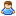1.You are currently browsing our forum as a guest. Create your own forum account to access all forum functionality.

# How to get the current rotor angle ?

Discussion in 'General' started by wagner, Oct 1, 2017.

This last post in this thread was made more than 31 days old.
1. In the program block, I am missing something or there is no "angle" attribute for the motors ( IMyMotorStator ) ?

Messages:
242
block.Angle

Careful on setting velocity with programming block. Last I checked the display is in a different scale than block.Velocity by about 10x.

I could not find this property, my IMyMotorBase dont have it, also I could not find anything in the docs, maybe its not there yet ?

Messages:
242
Last edited: Oct 2, 2017
•Agree x 1
5. ### w0lf3yApprentice Engineer

Messages:
152
I use ILSpy and it loads the game files when i use it, so it's always current. rotor.Angle is correct but it returns (I believe) radians.

here's small snippet i use to get a rotor's angle and read it's upper / lower limits then safty lock them (not shown) if it has reach it's limit.

Code:
```IMyMotorStator rotor = equipment[i] as IMyMotorStator;
Echo("Rotor Found: " + rotor.CustomName.ToString());
string rAngle = null;
string rAngleLength = null;
string rUpperLimit = null;
string rLowerLimit = null;
rAngleLength = MathHelper.ToDegrees(rotor.Angle).ToString();
string[] angleArray = rAngleLength.Split('.');
rAngle = angleArray;
Echo("Current Position: " + rAngle);
rLowerLimit = rotor.GetValue<float>("LowerLimit").ToString();
Echo("Lower Limit: " + rLowerLimit);
rUpperLimit = rotor.GetValue<float>("UpperLimit").ToString();
Echo("Upper Limit: " + rUpperLimit);```
the bits tha are probably most relevant to you are:
Code:
```rAngleLength = MathHelper.ToDegrees(rotor.Angle).ToString();
string[] angleArray = rAngleLength.Split('.');
rAngle = angleArray;```
I didn't write the special bits, I found them in a workshop code I tore apart, I would offer reference to original author, but it's been a long time and I've forgotten where i got it from.

6. ### PharapApprentice Engineer

Messages:
175
That's a very expensive way to extract the integer part from a float.

Two much cheaper options:
Code:
```float degrees = Math.Round(MathHelper.ToDegrees(rotor.Angle));
```
Code:
```int degrees = (int)MathHelper.ToDegrees(rotor.Angle);
```
Assuming of course you don't actually want the fractional part, otherwise
Code:
```float degrees = MathHelper.ToDegrees(rotor.Angle);
```
Is perfectly fine.